This node creates a half-sphere or semi-ellipsoid plot.

To add a Half-sphere node, right click in the Graph Editor and select Create Node  > Terrain Generation  > Half-sphere.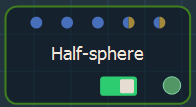Double click on the node to open its parameters: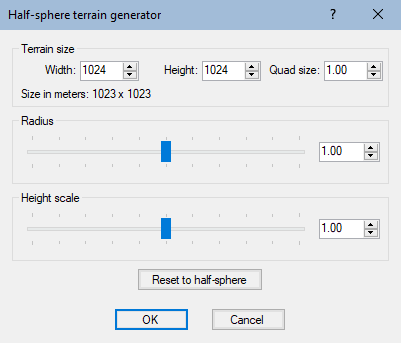Setting the terrain size

• To change the terrain size, edit the Width and Height fields in number of vertices.
• To change the quad size, edit the Quad size field in meters.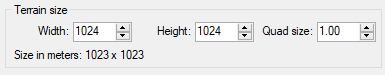Use the Radius slider to set the surface covered by the half-sphere: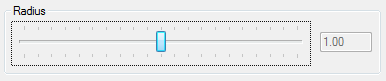• For a value of 1, the diameter of the half-sphere is exactly the size of the terrain, taking into account the smallest dimension of the terrain if it is not square.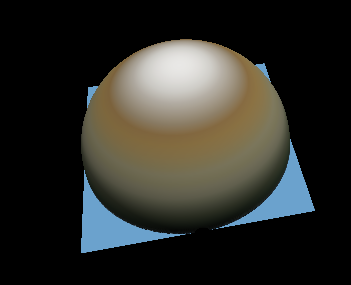• For a value less than 1, the half-sphere is contained entirely inside the terrain.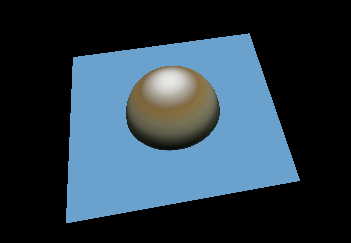• For a value greater than 1, the half-sphere is larger than the terrain.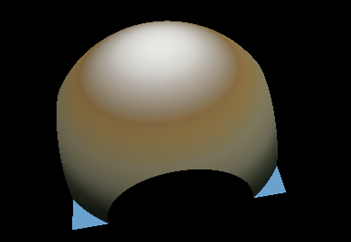Click OK to validate.

Setting the half-sphere height scale

Use the Height scale slider to set the maximum height of the terrain: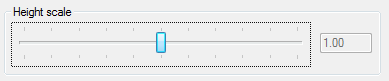• A value of 1 is the default value of the half-sphere (see above).
• For a value less than 1, the half-sphere is flattened.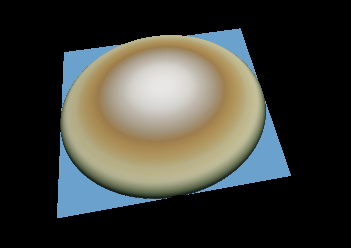• For a value greater than 1, the half-sphere is stretched.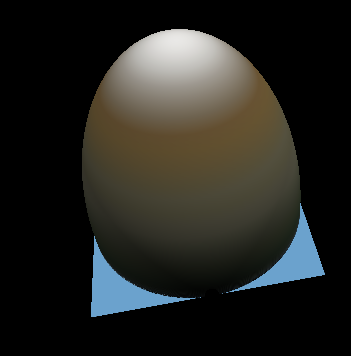Click OK to validate.

Working with connectors

The Half-sphere node has two connectors to drive a parameter using a formula or a mask.

• The first connector allows you to drive the radius of the half-sphere.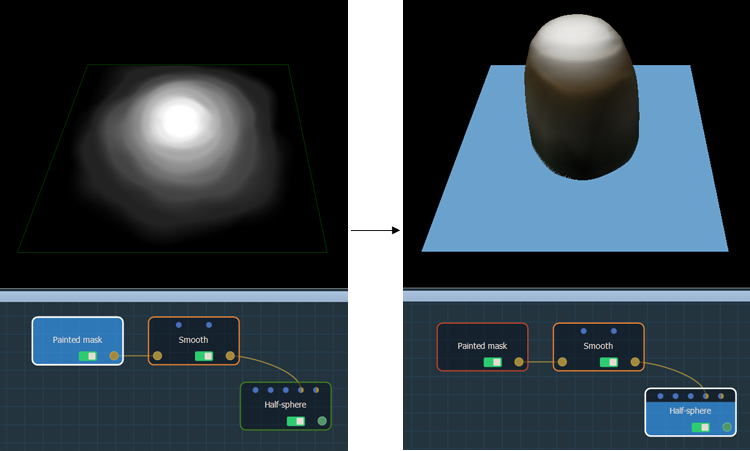• The second connector allows you to drive the height scale of the half-sphere.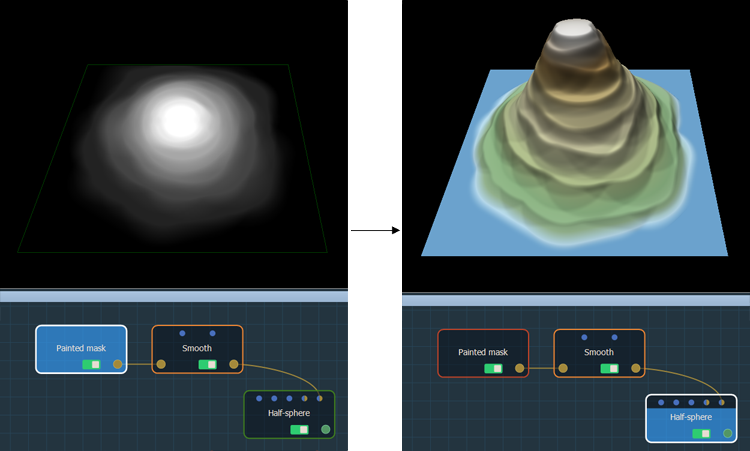Resetting the default values

The Reset to half-sphere button resets the half-sphere to the default values.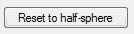Sample project

In the sample project below, two Slope terrain generators, one with a maximum height of 0 and the other with the inclination angle set to 200, are composed with a Full composition node.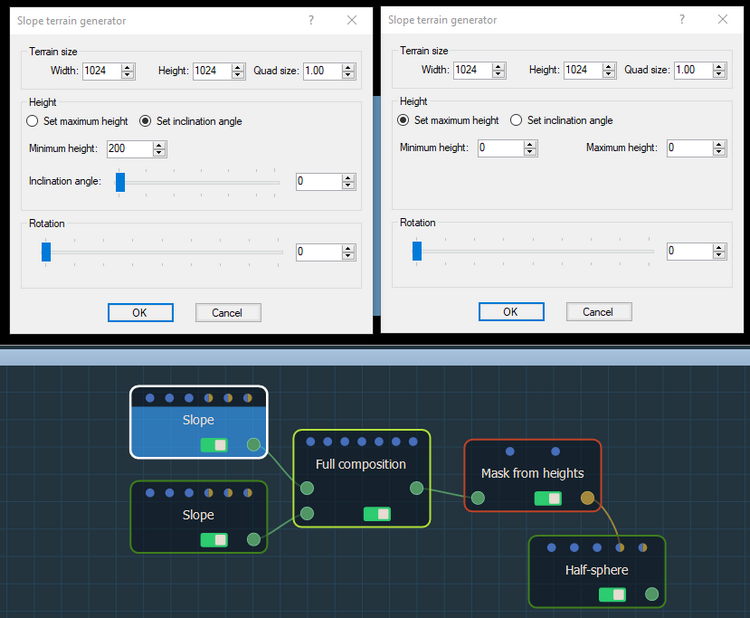The Full composition output is linked to a Mask from heights node, which has a gradient slope with a minimum height of 0 and a maximum height of 100.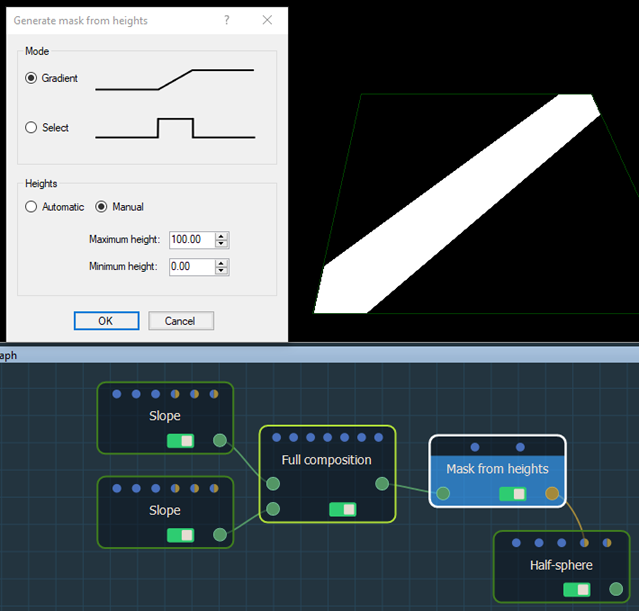The mask's output is linked to the Half-sphere's optional connector. The result is shown by clicking on the Half sphere node.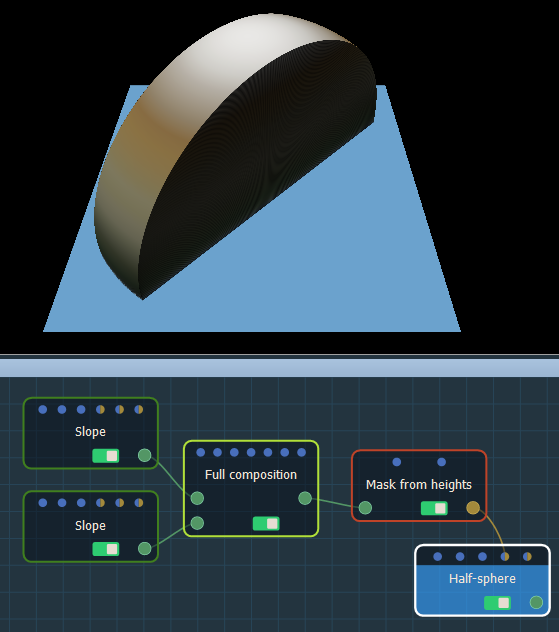Parameters

Parameter Use
Terrain size See Part common to all generator nodes
Radius Determines the surface covered by the half-sphere
Height scale Determines the maximum height of the terrain